## 22.1 Cube Root Algebra

In the same way that we found the F & D Series, which were able to represent square roots as patterns, we are now going to be deriving another F&D Series, which will represent cube roots.

### A. The Fraction Series for the Cube Roots

#### Same technique that we used to find square roots

Let us begin the same way we did with the square roots.First we let ‘X+1’ equal the cube root of any rational number. We then cube each side. We then expand the left side of the equation in the traditional way. We then subtract 1 from each side of the equation and factor out an X from the left side of the equation. In step 5 we let ‘c’ equal the constant on the right side of the equation. In step 6 for simplicity we replace ‘a/b - 1’ by ‘c’ and then divide both sides of the equation by the quadratic factor on the left. We end up with an expression for X as a function of itself.

#### X equals the cube root minus one

Remember from our ‘Given’ that we let ‘X + 1’ equal the cube root of any rational number, a/b. Thus X equals this cube root minus one. Shown below algebraically.### B. Beginning Bogus Equations or Going Nowhere

#### The easy way

First let us try the easy way. With our modern computers, iteration has become a tool that is easy to use. Remembering that X equals the cube root of some rational number minus one, let us just iterate this function in the following way to achieve the desired result.In this equation the most recent X, X(N) is a function of the X that went before, X(N-1). It is one of our usual contextual equations.

#### Computer testing

First we set up a simple computer program to iterate this function with a/b = 2, the simplest number because then c=1. Further we need to choose an X(0) to begin the iteration. Of course X(0) is the ‘seed’ of our iterated equation. We begin with the usual ‘0’ as our seed. Even more amazing than we expected, after 25 iterations are reached, our approximation has exceeded that of the computer; (accuracy greater than 17 decimals is beyond the capability of our computer). Then we vary the seed. No matter which seed we used, no matter how large or small, our iterated function consistently was unaffected, exceeding our computer capacity before 20 iterations were reached. Even X(10), the tenth iteration was little affected by changing the seed value.

#### Simple! but boring

Wow! This was simple, but boring. Similarly we could use the same technique to generate fourth roots, fifth roots and the like. This is the result we were looking for. Common family for irrationals and rationals. End of story; end of paper. So what? So much simplicity that the results are irrelevant, meaning very little.

#### Works well 2 and under

However before going on down to the beach, let us try some other values besides 2 for our rational number, a/b. Let us not be too hasty. We try values under 2. They all work very easily, although a little more slowly. When our rational number is ‘1’, everything turns to zero, which is what we expect. When the rational number that we are finding the Root of is under one the iterations approach the computer accuracy more slowly, but they still approach the desired result. Still the seed seems to have no effect. Break out the suntan lotion, here we come. All we have to do now is to check a few rational numbers over 2 to exhibit our trivial truth.

#### Over 2: Uh! Oh! Bifurcation problems

First we call our rational number, 3; our iterative function approaches its Root with no problem. Then 4, still no problems. Suntan here we come. Let’s try 10. Our iterated function is approaching the cube root more and more slowly. How about 100? Oops! Dropped the ball. Our function generates a distinct bifurcation that sets up relatively early. One gets two distinct results, neither of which is correct although one closer than the other. One is off by over 20 the other by about 4, a range of about 30. This iteration does not work at all. In looking more closely at the rational numbers that our iterative function will generate the cube root of, we find that when our rational number is only 22 that our function begins to bifurcate slightly, with a range of about 2.

#### No family of numbers with this style equation

Thus our iterative equation works well when our rational number is constrained. It works well when our rational number is 2 and under. However as our rational number begins to rise, more and more iterations are necessary to approach the desired limit. Then when our rational number is 22; the limit of our iterated functions bifurcates, neither one the desired answer. The answers become less and less correct as our rational number rises. Hence our iteration works exceptionally well within constrained values for our rational number that we are finding the cube root of. However when the rational number grows to even 22, the iteration bifurcates inaccurately. This is unacceptable. Our family of numbers certainly can’t exclude all numbers above 22, to be at all significant. Put away the beach umbrella and back to work. (We will deal more thoroughly with this class of equations in another Notebook called Root Beings in a section called ‘Stressed out Equations’, i.e. equations that work well under normal circumstances, but who break down under stress and why.)

### C. Another method of iteration

Let us look a little more closely at how our original equation, X as a function of itself, an iterated function, actually works, by sinking into its bowels to explore a different method of iteration.

#### Rules for iteration

Instead of iterating both X’s of our equation simultaneously, as we did previously, let us only iterate one at a time. We will always iterate the left most X first. Thus we will generate a series of X functions, each of which is successively more complicated. X(0) equals X itself, while X(1) equals the iteration equation, shown below again because of its importance.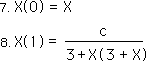Iterating the left most X we generate X(2). Iteration in this case means substituting the expression for X(1) for the left most X in the equation.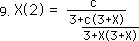In our expression for X(2) we have two left-most X’s, which we expand or iterate to get X(3). Shown below.#### What does it mean? More complexity

What do all of these iterations mean? We have only done three and already our equation is incredibly confusing. Besides all of X functions equal the same thing, no matter how far they are iterated, they are all approximations of the cube root of the same rational number. These equations certainly don’t simplify any computations. What next? The Return of the celebrated F Series that proved so useful and fascinating when dealing with square roots.

### D. A series of fractions or the F Series

#### Generating ƒ(1) -> ƒ(3)

Generating ƒ(1) -> ƒ(3) we will create a series of fractions whose limit is our desired cube root. Each fraction is based upon a corresponding X function. While the X functions all equal the cube root, each of the ƒ fractions is only an approximation. There is only one rule for turning an X function into a ƒ fraction. Treat each X in the X functions as a zero. Applying this strategy to X(0), ƒ(0) = 0. Similarly X(1) becomes ƒ(1) = c/3. Below are the first few ƒ fractions based upon the corresponding X functions.In step 12 we noticed that c/3, which equals ƒ(1), was contained in the expression for ƒ(2). In anticipation of future results we substituted ƒ(1) for c/3 to get the right most expression for ƒ(2). In step 13 we performed a similar substitution twice for ƒ(3). In the right-most expression in step 13 we noticed the expression for ƒ(2) contained in ƒ(3)’s denominator. Thus in step 14 we substitute ƒ(2) for this expression.

#### Rules for Generating the X and ƒ functions

We can now generate some more X functions and ƒ fractions using the same rules we mentioned above. They are roughly stated below.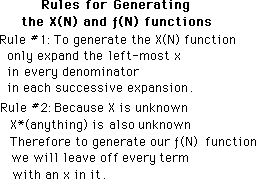#### Rules for expansion

The expansion of the left most X changes it from an X(0) to an X(1) by definition. For simplification we will use that terminology in the future expansion. In general an expansion of an X function means to turn it from X(N) to X(N+1), shown below.#### The X(4) function and the value of ƒ(4)

Using the above rules and notation, we generate X(4), shown below. We just replaced the X’s to be expanded with an X(1) for simplification.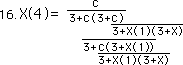Remembering that ƒ(1) = c/3 is an approximation of X(1) and that ƒ(0) = 0 is an approximation of X(0) =X, we substitute these expressions into X(4) to obtain the value for ƒ(4), shown below.In the middle of the expression we notice the expression for ƒ(2) from step 12. We substitute ƒ(2) for it in the right most expression. We see the same expression for ƒ(2) at the bottom of the expression for ƒ(4). We also replace it with ƒ(2) below.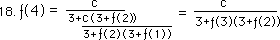Finally we notice the expression for ƒ(3) from step 13 in the denominator of ƒ(4) and substitute ƒ(3) for it in the right most expression.

#### X(5) and ƒ(5)

By the same rules we can generate X(5) from X(4). Each X(1) becomes an X(2) and each left X becomes X(1).From X(5), ƒ(5) can be generated, by simply substituting ƒ(2) for X(2) and ƒ(1) for X(1).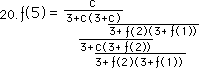Remembering the expression for ƒ(3) we can make two more substitutions to achieve the following result.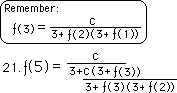Again we notice the expression for ƒ(4) in our equation. We substitute ƒ(4) in the equation to achieve our final simplification.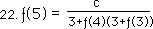#### Towards a general expression for the X and ƒ series

Remembering that the ƒ fractions are only approximations of the X functions. We express equation 22 in terms of the X functions.Remembering that X(N) expands into X(N+1), we easily generate X(6). The pattern is obvious enough to generate an expression for X(N). While all of the X series no matter how little or great N is, all equal the cube root of the rational number, a/b, minus 1, the ƒ series gives us the approximation. Substituting the approximate ƒ function for the exact X function, we get the following general formula for the ƒ series.#### Capital F notation

Remembering the F(R) notation from our last paper, we rewrite this equation with another notation.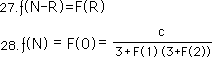#### ƒ(N) approximates the Cube Root minus one

Remember that as N approaches infinity, ƒ(N) approaches X(N). We will also use the ƒ(∞) terminology developed in the last paper.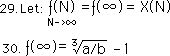What this expression basically says is: as the number of iterations of Equation 25 approaches infinity that the iterated X approaches our desired cube root minus one. We call it a ƒ fraction instead of the X function only to imply its approximate value.

#### Computer testing

Let us check our work, especially considering the fiasco of our first iterated function. On the computer we iterate equation 25 or 26, (they are essentially the same), with a variety of numbers, small and large, and the value always approaches the desired limit. As with our first iterated function, the limit is approached rapidly with numbers under 10. However while our first iterated function bifurcated after 22, this one slowly approaches the limit no matter how large the number that we are finding the cube root of. As an example: Given that our rational number is 10,000, after 250 iterations the approximation for our derived Equation #25 is within 1/100,000th of the cube root, while the bifurcations of our first iterated function are alternately off by 20 and 3000.

### E. The Denominator Series for Cube roots

#### History

In our discussions of square roots we introduced the Denominator Series, the D Series. There are a few interesting aspects to this D Series that are worth noting in connection with this section. First: The value of each member is a function of the two previous members of the series. Second: If the seed, X(0), of the series is a whole number then all the members of the series are also whole numbers. Third, and most importantly for this discussion, the ratio of the consecutive elements of the Denominator Series approaches distinct square roots. Finally, the value of the seed, X(0), has virtually no effect upon this ratio, except when it equals zero, in which case it neutralizes the series. To indicate the relative importance of this series, the Fibonacci sequence is a special case of this Denominator Series for square roots.

#### Assume there exists a D Series for cube roots

In this section on cube roots, we would like to find a Denominator Series for cube roots. Let us start with the assumption that it exists.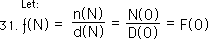#### ‘Cap D’ vs. ‘small d’ notation

Note that two different notational systems are shown. Both have their advantages and disadvantages and will be used relatively interchangeably. The basic idea is that d(N-R)=D(R). The ‘small d’ system implies that one started at zero, has reached N, and is moving forward, being continuously affected in a contextual environment, by what has just passed. The large D notational system implies that one exists in the Now and is affected by the most recent layers of sep-R-ation from this spot. They are both right in the proper context.

#### Substitute into F Series for Cube Roots

We substitute these numerator and denominator expressions into Equation 26 for the function expression on the right hand side of the equation.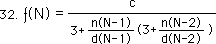For simplification we write the same expression in the ‘cap D’ system, derived from Equation 28.Using elementary algebra, we simplify the expression, turning it into an ordinary fraction, without multiple levels.#### Numerator a function of the denominator

We notice that the Numerator series is a function of the Denominator series, written below.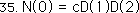We then substitute this expression for the numerator expressions in equation 34, obtaining the following.D(2) is a common factor in the numerator and the denominator of the fraction. We factor it out and cancel it out, giving us the following expression.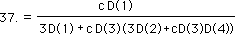#### Delta, ∆, Notation

Ultimately we want to write our D series in whole numbers only. Therefore let us convert the constant ‘c’ into its whole number equivalent. This is shown below with the delta, ∆, representing the difference between the numerator and the denominator, a-b.We then turn Equation 37 into an expression that contains only whole numbers in the top and bottom of the fraction.The ∆D(1) of the numerator is the same expression that we derived for the numerator of the square root series. However both the numerator and denominator of this expression have already been changed by factoring out and canceling out the D(2) expression. Thus the numerator series that this represents is different from our original numerator series and so will be denoted by N’(0), shown below.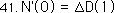Let us assume for purposes of experimentation, that a numerator and denominator series for cube roots exists that has this type of relation. We begin in the same way we did in Equation 33, substituting the N’ and D’ Series for the F series of Equation 28. This is shown below.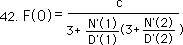We then substitute the ∆D’ expressions for the N’ expressions, in equation 43.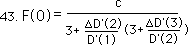Using simple algebra, we combine terms, canceling out the D’(2) of the denominator, combining terms again, and then finally flipping the denominator of the denominator into the numerator to obtain the simple fraction, shown below.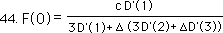We then convert to the delta terminology so that our numerator and denominator are whole numbers. Below is our result.#### General expression for the Denominator Series for Cube Roots

Note that the ∆D’(1) that we assumed to be the N’ numerator series, is indeed in the numerator position. Thus our assumption was correct. We can now write an expression for the Denominator Series for cube roots.### F. The Three Basic Iterative Theorems for Cube Roots

Now we can state our three basic iterative Theorems for Cube Roots. These are the F Series Theorem, the Numerator Theorem and the Denominator Theorem, i.e. the First, Second and Third Theorems in that order. While these Theorems have not been thoroughly established deductively, they have been tested under a variety of circumstances always yielding the cube roots of the appropriate numbers. The likelihood of ‘accidentally’ coming up with the cube root of any number is so unlikely that we are safe in saying that these Theorems for cube roots have been conclusively verified experimentally.

#### The F Series Theorem for cube roots, the First Theorem

Let us begin with the First Theorem, the F Series for cube roots.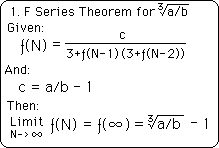This theorem states the second order iterative expression, i.e. two layers of feedback, which the F Series is based upon. It then points out that the Limit of this iteration, i.e. as the number of iterations approaches infinity, is the Cube Root of our rational number, a/b, minus one.

#### Numerator Theorem for cube roots identical to that for square roots

The Second Iterative Theorem for cube roots, the Numerator Theorem is shown below. It is identical to the Numerator Theorem for square roots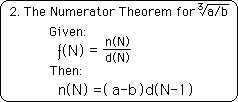#### Denominator Theorem for Cube Roots

The Third Iterative Theorem has to do with the relation between the F & D Series. It is called the Denominator Theorem. The Third Theorem for cube roots is shown below.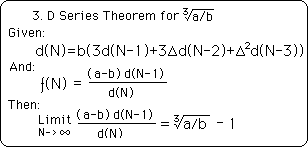Note that while the iterative expressions for the D Series is different for cube and square roots that the relation of the D Series to the F Series is identical.

### G. Comparisons and parallels between cube and square root theorems

Let us now compare and contrast the F, D, and N Series for cube and square roots. Then we will finish this section by looking at some of the properties of our System.

#### In Circle Notation

For simplification let us write the D Series in the circle notation.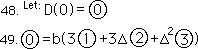Simply speaking each member of the D Series for cube roots is a function of the three elements of the series that went before.

#### Subscript notation to differentiate the order of the F or D Series

To distinguish between the different D and F series and their different roots we will introduce a subscript.D and F Series of the top line refer to square roots, the D and F Series of the middle line refer to cube roots and the D and F Series of the last line refer to Nth roots. This subscript also works with the N Series and the ‘small d’ style notation. Further, for ease of notation, unless noted otherwise, once the level of F, D or N series is noted, all others in the equation are of the same level.

#### D2 Series relies on the past two, D3 relies on the past three

Now we can write the D series for square roots with the circle notation for comparison.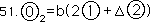Note that while the elements of the D Series for cube roots are a function of the past three elements of the series that the elements of the D Series for square roots are only a function of the last two elements.

#### Parallels with the D series for square roots

Below are the general expressions for the F Series in circle notation.Note that the numerator in both cases is a product of the past denominator multiplied by delta, ∆=a-b.#### The root of 2, the simplest expression

The simplest form of the D & F Series is when the Root Number, a/b, equals 2.

If our rational number is 2, then the ∆ term becomes 1. Then our equations for the N, D & F Series simplifies to the following set of equations.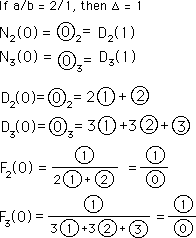#### Numerator is the past Denominator if the Root Number is 2

Note that the past denominator is the present numerator. This is the only D Series where the ratio of the consecutive elements of series approaches the square root. With all the rest of the rational numbers it is the ratio of the consecutive elements multiplied by delta, ∆=a-b, which approaches the Root.

#### The Bogus F Series

At this rational number, i.e. 2, we will see that many forms of the Fraction and Denominator Series approach the Root. However when ‘a’ grows large compared to ‘b’ then the ∆ term perturbs the equation enough to distort the result from all but the equations listed above. This was the case with our initial iterated function, which bifurcated when greater than 22. It is shown below. We will call it the Bogus F Series, because it looks similar to the Real F Series. It also works like the Real F Series under ‘normal’ conditions. However the Bogus F Series breaks down under ‘extreme’ conditions while the Real F Series continues to do the work of approximating the Root.When our rational number is 2, then c equals 1 and our equation works quite well for approximating the Root of 2. However as ‘a’ grows large compared to ‘b’ then ‘c’ also grows large. When ‘c’ is relatively small the Bogus F Series works quite well. However as ‘c’ grows the Bogus F Series works more and more poorly until it finally splits apart, bifurcating to give us a meaningless result.

#### Bogus F Series works well when our rational number, a/b, is between 2 and 0.

Another feature to these F&D equations is that as ‘b’ grows large compared to ‘a’, i.e. when the rational number, a/b, approaches zero, then c approaches ‘-1’. Remember that ‘c’ is the numerator of the F Series. (c = a/b-1 = (a-b)/b, where ‘a/b’ is the rational number that we desire the Root of.) Thus as a/b goes from 2 to 0, c goes from 1 to -1, reaching 0 when a/b = 1. Because this coefficient to the F series stays within such small limits, the bogus F Series, works well when a/b is between 2 and 0.

#### Zero is the only exact number in the F Series

Another thing to note concerning these series is that when a/b=1 then ∆ = a-b = 0. If ∆ = 0 then the F series equals zero, exactly. This is the only number in the F series that is exact. All the rest of the numbers are approximations. As the number of iterations approaches infinity the aforementioned ratio approaches the desired root, but it is never exact. Hence zero, nothing, emptiness, is the only exactly precise number in our extended family of numbers, represented by our F & D Series.

#### Seeds can’t all be zero for the D Series

We’ve mentioned that the seed is secondary to the pattern established by the D Series. Basically the seed, X(0), has virtually no influence on the Root Ratio. However because it is a Denominator Series, it cannot equal zero. Therefore the root seed cannot be all zeros. Thus the D Series for square roots actually has 2 seeds because it depends upon the past two numbers to generate the next value. While either of the seeds can be zero, both cannot. If both equal zero then the Denominator Series becomes zero and our F Series, which determines the Root, becomes undefined. Similarly for the cube root formulas: the D Series for cube roots has three seeds. Any of these seeds may be zero without having any effect upon the Root, while if all three seeds are zero, then the D Series turns to zero and then the F Series is undefined. Hence the seeds can be any value, with the one exception that they cannot all be zero. (Note this is to be contrasted with the F Series where an all zero seed group is no problem at all.)

#### Moving on to fourth roots

Now that we have finished with the F&D Series for cube roots let us move on to the fourth roots. We don’t know what will happen. Read on to find if there are any iterative systems that yield up the fourth roots.